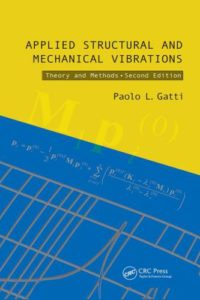اسم المؤلف
Paolo L.Gatti and Vittorio Ferrari
التاريخ
2 نوفمبر 2018
المشاهدات
التقييمLoading...

Applied Structural and Mechanical Vibrations
Theory, methods and measuring instrumentation
Paolo L.Gatti and Vittorio Ferrari
Contents
Preface
Acknowledgements
PART I
Theory and methods
P.L.GATTI
1 Review of some fundamentals
1.1 Introduction
1.2 The role of modelling (linear and nonlinear, discrete
and continuous systems, deterministic and random data)
1.3 Some definitions and methods
1.4 Springs, dampers and masses
2 Mathematical preliminaries
2.1 Introduction
2.2 Fourier series and Fourier transforms
2.3 Laplace transforms
2.4 The Dirac delta function and related topics
2.5 The notion of Hilbert space
References
3 Analytical dynamics—an overview
3.1 Introduction
3.2 Systems of material particles
3.3 Generalized coordinates, virtual work and d’Alembert principles:
Lagrange’s equations
3.4 Hamilton’s principle of least action
3.5 The general problem of small oscillations
3.6 Lagrangian formulation for continuous systems
References
4 Single-degree-of-freedom systems
4.1 Introduction
4.2 The harmonic oscillator I: free vibrations
4.3 The harmonic oscillator II: forced vibrations
4.4 Damping in real systems, equivalent viscous damping
References
5 More SDOF—transient response and approximate methods
5.1 Introduction
5.2 Time domain—impulse response, step response and convolution
integral
5.3 Frequency and s-domains. Fourier and Laplace transforms
5.4 Relationship between time-domain response and
frequency-domain response
5.5 Distributed parameters: generalized SDOF systems
References
6 Multiple-degree-of-freedom systems
6.1 Introduction
6.2 A simple undamped 2-DOF system: free vibration
6.3 Undamped n-DOF systems: free vibration
6.4 Eigenvalues and eigenvectors: sensitivity analysis
6.5 Structure and properties of matrices M, K and C: a few
considerations
6.6 Unrestrained systems: rigid-body modes
6.7 Damped systems: proportional and nonproportional
damping
6.8 Generalized and complex eigenvalue problems: reduction to
standard form
References
7 More MDOF systems—forced vibrations and response analysis
7.1 Introduction
7.2 Mode superposition
7.3 Harmonic excitation: proportional viscous damping
7.4 Time-domain and frequency-domain response
7.5 Systems with rigid-body modes
7.6 The case of nonproportional viscous damping
7.7 MDOF systems with hysteretic damping
7.8 A few remarks on other solution strategies: Laplace transform
and direct integration
7.9 Frequency response functions of a 2-DOF system
References
8 Continuous or distributed parameter systems
8.1 Introduction
8.2 The flexible string in transverse motion
8.3 Free vibrations of a finite string: standing waves and
normal modes
8.4 Axial and torsional vibrations of rods
8.5 Flexural (bending) vibrations of beams
8.6 A two-dimensional continuous system: the flexible membrane
8.7 The differential eigenvalue problem
8.8 Bending vibrations of thin plates
8.9 Forced vibrations and response analysis: the
modal approach
8.10 Final remarks: alternative forms of FRFs and the introduction
of damping
References
9 MDOF and continuous systems: approximate methods
9.1 Introduction
9.2 The Rayleigh quotient
9.3 The Rayleigh-Ritz method and the assumed modes method
References
10 Experimental modal analysis
10.1 Introduction
10.2 Experimental modal analysis—overview of the
fundamentals
10.3 Modal testing procedures
10.4 Selected topics in experimental modal analysis
References
11 Probability and statistics: preliminaries to random vibrations
11.1 Introduction
11.2 The concept of probability
11.3 Random variables, probability distribution functions and
probability density functions
11.4 Descriptors of random variable behaviour
11.5 More than one random variable
11.6 Some useful results: Chebyshev’s inequality and the central limit
theorem
11.7 A few final remarks
References
12 Stochastic processes and random vibrations
12.1 Introduction
12.2 The concept of stochastic process
12.3 Spectral representation of random processes
12.4 Random excitation and response of linear systems
12.5 MDOF and continuous systems: response to random
excitation
12.6 Analysis of narrow-band processes: a few selected topics
References
PART II
Measuring instrumentation
V.FERRARI
13 Basic concepts of measurement and measuring instruments
13.1 Introduction
13.2 The measurement process and the measuring instrument
13.3 Measurement errors and uncertainty
13.4 Measuring instrument functional model
13.5 Static behaviour of measuring instruments
13.6 Dynamic behaviour of measuring instruments
13.8 Performance specifications of measuring instruments
13.9 Summary
References
14 Motion and vibration transducers
14.1 Introduction
14.2 Relative- and absolute-motion measurement
14.3 Contact and noncontact transducers
14.4 Relative-displacement measurement
14.5 Relative-velocity measurement
14.6 Relative-acceleration measurement
14.7 Absolute-motion measurement
14.8 Accelerometer types and technologies
14.9 Accelerometer choice, calibration and mounting
14.10 General considerations about motion measurements
14.11 Force transducers
14.12 Summary
References
15 Signal conditioning and data acquisition
15.1 Introduction
15.2 Signals and noise
15.3 Signal DC and AC amplification
15.4 Piezoelectric transducer amplifiers
15.5 Noise and interference reduction
15.6 Analogue-to-digital conversion
15.7 Data acquisition systems and analysis instruments
15.8 Summary
References
Appendices
P.L.GATTI
A Finite-dimensional vector spaces and elements of matrix analysis
A.1 The notion of finite-dimensional vector space
A.2 Matrices
A.3 Eigenvalues and eigenvectors: the standard eigenvalue
problem
A.4 Matrices and linear operators
References
B Some considerations on the assessment of vibration intensity
B.1 Introduction
B.2 Definitions
References